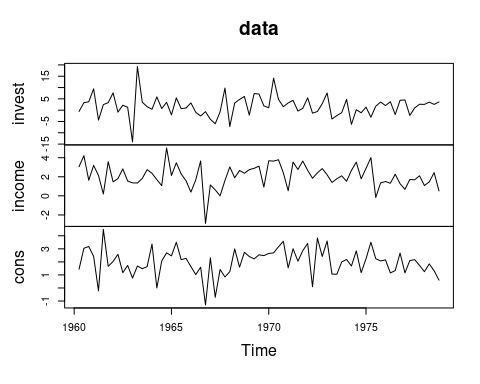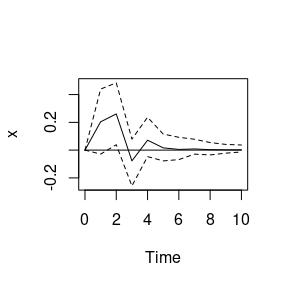# Model Comparison in bvartools

#### 2023-08-30

bvartools comes with the functionality to set up and produce posterior draws for multiple models in an effort to reduce the time required for this potentially laborious process. This vignette illustrates how the package can be used to set up multiple models, produce prior specifications, obtain posterior draws and select the model with the best fit in a few steps.

## Data

For this illustrations the data set E1 from Lütkepohl (2006) is used. It contains data on West German fixed investment, disposable income and consumption expenditures in billions of DM from 1960Q1 to 1982Q4. Like in the textbook only the log-differenced series up to 1978Q4 are used.

library(bvartools)

set.seed(123456) # Set seed for reproducibility

data <- diff(log(e1)) * 100 # Obtain log-differences

# Use date up to 1978Q4
data <- window(data, end = c(1978, 4))

# Plot
plot(data)## Set up models

Functions gen_var can be used to obtain a list of different model specifications. In the following example five models with an intercept and increasing lag orders are generated.

object <- gen_var(data, p = 0:4,
deterministic = "const",
iterations = 5000, burnin = 1000)

All objects use the same amounts of available observations to ensure consistency for the calculation of information criteria for model selection.

## Priors

Function add_priors can be used to produce priors for each of the models in object models.

object <- add_priors(object,
coef = list(v_i = 0, v_i_det = 0),
sigma = list(df = "k", scale = 0.0001))

## Estimation

Posterior draws can be obtained using function draw_posterior. The function allows to specify the number of CPUs, which are available for parallel computing.

object <- draw_posterior(object, mc.cores = 3)

If multiple models are estimated the function produces an object of class bvarlist, which is a list of objects of class bvar. Thus, each element of the list can be used for further analysis.

## Model selection

If function summary is applied to an object of class bvarlist, it produces a table of information criteria for each specification. The information criteria are calculated based on the posterior draws of the respective model and calculated in the following way:

• Log-likelihood: $$LL = \frac{1}{R} \sum_{i = 1}^{R} \left( \sum_{t = 1}^{T} -\frac{K}{2} \ln 2\pi - \frac{1}{2} \ln |\Sigma_t^{(i)}| -\frac{1}{2} (u_t^{{(i)}\prime} (\Sigma_t^{(i)})^{-1} u_t^{(i)} \right)$$ for each draw $$i$$ and $$u_t = y_t - \mu_t$$;
• Akaika information criterion: $$AIC = 2 (Kp + M (s + 1) + N) - 2 LL$$;
• Bayesian information criterion: $$BIC = ln(T) (Kp + M (s + 1) + N) - 2 LL$$;
• Hannan-Quinn information criterion: $$HQ = 2 ln(ln(T)) (Kp + M (s + 1) + N) - 2 LL$$.

$$K$$ is the number of endogenous variables and $$p$$ the lag order of the model. If exogenous variables were used $$M$$ is the number of stochastic exogenous regressors and $$s$$ is the lag order for those variables. $$N$$ is the number of deterministic terms.

summary(object)
#>   p s        LL      AIC      BIC       HQ
#> 1 0 0 -420.5955 843.1909 845.4536 844.0907
#> 2 1 0 -413.5161 835.0323 844.0830 838.6314
#> 3 2 0 -405.9250 825.8500 841.6887 832.1485
#> 4 3 0 -408.6642 837.3284 859.9552 846.3264
#> 5 4 0 -406.7913 839.5825 868.9974 851.2799

Since all information criteria have the lowest value for the model with $$p = 2$$, the third element of object is used for further analyis.

plot(irf(object[], impulse = "income", response = "cons", n.ahead = 10))## Literature

Chan, J., Koop, G., Poirier, D. J., & Tobias, J. L. (2019). Bayesian Econometric Methods (2nd ed.). Cambridge: University Press.

Lütkepohl, H. (2006). New introduction to multiple time series analysis (2nd ed.). Berlin: Springer.